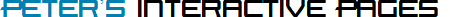CalculationController Controls

The CalculationController lets you describe calculations that involve numbers in textboxes, constants and other logic. The values from these calculations can be used in the following ways:
• Displayed on the page, whether in a Label or a textbox.
• Validators that compare numbers can evaluate the value simply by setting their ControlIDToEvaluate property to this control's ID. Supported validators include: CompareToValueValidator, CompareTwoFieldsValidator, RangeValidator, and DifferenceValidator. In addition, the RequiredTextValidator can determine if the calculation had an error.
• Like Validators, their Conditions can evaluate the value. For example, the Enabler property on various controls use Conditions. Now those Conditions can enable their control based on the result of a calculation.
• The calculations can include the values of other CalculationControllers on the page. This allows reuse of a common calculation, reducing the size of the client-side code and slightly improving performance by reducing the number of times the code executes a calculation.
While it is typical to add together the values of textboxes to form a total, the CalculationController can handle far more powerful expressions. Here are the elements that you can use to develop your expressions:
• Use these textboxes: IntegerTextBox, DecimalTextBox, CurrencyTextBox, and PercentTextBox.
• Lists, DropDownLists, RadioButtonLists, and CheckBoxLists can have numeric values assigned to each selectable element that are used when selected.
• Checkboxes and RadioButtons can have numeric values for their checked and uncheck states.
• Constants (numbers)
• Subexpressions which are the same idea as putting parenthesis around an expression to have it calculate together.
• Use any Condition object to select between two expressions. One runs if the Condition evaluates as "success". The other, if the Condition evaluates as "failed". This allows your expression to have different logic based on the settings on the screen. Basically, you are developing "IF" statements.
Since the CompareToValueCondition and RangeCondition now can evaluate the values of CalculationControllers, your IF statements can be decided by calculations too.
• Each element – textbox, constant, subexpression, and "IF" statement – can use these operators: add, subtract, multiply, and divide.
Here is an example of two CalculationControllers on a page, using the image shown in design mode. They refer to three controls: two DecimalTextBoxes and a CheckBox. The DecimalTextBoxes are used for the calculation. The CheckBox is used for the Condition object (a CheckStateCondition):To further refine your expressions, the CalculationController has these features:
• The result, which is initially a decimal value, can be rounded in several ways. It can round to a certain number of decimal places and use different rounding rules.
• For blank textboxes, you can determine if its an error or treated as 0.
• You can supply client- and server-side functions that let you customize the result of any element. For example, if you want the value of a textbox to be run through the Sin() function, you write a function to use that calculation. The CalculationController will pass you the value. Your function can report an error for an illegal value and correct errors.
• It gracefully handles errors, such as divide by zero.

### Demo 1 - The Sum of Three DecimalTextBoxes

These three DecimalTextBoxes are added together and the result is shown in a label to the right.
This demo shows "n/a" in the Label if any of the textboxes are blank, although you have the option to treat blank textboxes as 0 too.
A RangeValidator is assigned to the CalculationController to report an error if the sum is not between 0 and 100. A RequiredTextValidator is assigned to the CalculationController to report when the calculation failed (due to a blank textbox or illegal text).

= 0.0

### ASP.NET Syntax for this demo

```<des:DecimalTextBox id="DecimalTextBox1" runat="server">0</des:DecimalTextBox>
<des:DecimalTextBox id="DecimalTextBox2" runat="server">0</des:DecimalTextBox>
<des:DecimalTextBox id="DecimalTextBox3" runat="server">0</des:DecimalTextBox>
=
<asp:Label id="Label2" runat="server">Label</asp:Label>
<des:RequiredTextValidator id="RequiredTextValidator1" runat="server"
ControlIDToEvaluate="CalculationController1" ErrorMessage="Please assign valid numbers to all textboxes">
<ErrorFormatterContainer>
<des:TextErrorFormatter Display="Dynamic" />
</ErrorFormatterContainer>
</des:RequiredTextValidator>
<des:RangeValidator id="RangeValidator1" runat="server"
ControlIDToEvaluate="CalculationController1"
ErrorMessage="The total must be in the range of {MINIMUM} and {MAXIMUM}."
DataType="Double" Minimum="0" Maximum="100">
<ErrorFormatterContainer>
<des:TextErrorFormatter Display="Dynamic" />
</ErrorFormatterContainer>
</des:RangeValidator><br>
<des:CalculationController id="CalculationController1" runat="server"
ShowValueControlID="Label1" InvalidValueLabel="n/a" InvalidValueCssClass="DESVALErrorText"
ValidateOnCalc="True" AutoShowValue="Always">
<Expression>
<des:NumericTextBoxCalcItem BlankIsZero="False" TextBoxControlID="DecimalTextBox1" />
<des:NumericTextBoxCalcItem BlankIsZero="False" TextBoxControlID="DecimalTextBox2" />
<des:NumericTextBoxCalcItem BlankIsZero="False" TextBoxControlID="DecimalTextBox3" />
</Expression>
</des:CalculationController>```

### Demo 2 - Using Conditional Logic

Suppose this is a checkout page where you have placed an order. You enter the quantity, which is multiplied by the price.
In addition, you use a DropDownList to select between one of three shipping rates.
There are 3 CalculationControllers here. The first calculates the product price x quantity. It updates row 1.
The second row uses a CalculationController to select between several possible prices and display its value.
The third row uses a CalculationController to add the value of the first and second rows.

 Product name Price Quantity Total Computer Desk #5312-30 \$200.00 \$200.00 Shipping Overnight 3 - 5 days Standard Ground \$12.80 Total Price \$212.80

### ASP.NET Syntax for this demo

```<table style="width:500px;">
<tr class='TableTitles'>
<td style="width:200px;">Product name</td>
<td style="width:100px;">Price</td>
<td style="width:100px;">Quantity</td>
<td style="width:100px;">Total</td>
</tr>
<tr class="TableRows">
<td>Computer Desk #5312-30</td><td align=right>\$200.00</td>
<td><des:IntegerTextBox id="ProductQty1" runat=server Width="30px"
ShowSpinner="True" SpinnerMinValue="1" SpinnerMaxValue="99" AllowNegatives="False">1</des:IntegerTextBox></td>
<td align=right>
<des:CalculationController id="ProductCalc1" runat=server
ShowValueControlID="ProductTotal1" LabelFormatCurrencySymbol="True" LabelFormatThousandsSep="True"
DecimalPlaces="Currency" AutoShowValue="Always">
<Expression>
<des:ConstantCalcItem Constant="200" />
<des:NumericTextBoxCalcItem TextBoxControlID="ProductQty1" Operator="Multiply" />
</Expression>
</des:CalculationController>
<asp:Label ID="ProductTotal1" Runat=server></asp:Label>
</td>
</tr>
<tr class=TableRows>
<td>Shipping
<asp:DropDownList ID="ShippingDDL" Runat=server>
<asp:ListItem Value="Overnight">Overnight</asp:ListItem>
<asp:ListItem Value="3 - 5 days">3 - 5 days</asp:ListItem>
<asp:ListItem Value="Standard Ground">Standard Ground</asp:ListItem></asp:DropDownList></td>
<td> </td><td> </td>
<td align=right>
<des:CalculationController id="ShippingCalc" runat=server
ShowValueControlID="ShippingTotal" LabelFormatCurrencySymbol="True" LabelFormatThousandsSep="True"
DecimalPlaces="Currency" AutoShowValue="Always">
<Expression>
<des:ConditionCalcItem>
<ConditionContainer>
<des:SelectedIndexCondition ControlIDToEvaluate="ShippingDDL" />
</ConditionContainer>
<ExpressionWhenTrue>
<des:ConstantCalcItem Constant="12.8" />
</ExpressionWhenTrue>
<ExpressionWhenFalse>
<des:ConditionCalcItem>
<ConditionContainer>
<des:SelectedIndexCondition ControlIDToEvaluate="ShippingDDL" Index="1" />
</ConditionContainer>
<ExpressionWhenTrue>
<des:ConstantCalcItem Constant="8.75" />
</ExpressionWhenTrue>
<ExpressionWhenFalse>
<des:ConstantCalcItem Constant="4.25" />
</ExpressionWhenFalse>
</des:ConditionCalcItem>
</ExpressionWhenFalse>
</des:ConditionCalcItem>
</Expression>
</des:CalculationController>
<asp:Label ID="ShippingTotal" Runat=server></asp:Label>
</td>
</tr>
<tr><td><b>Total Price</b></td><td> </td><td> </td>
<td align=right>
<des:CalculationController id="TotalCalc" runat=server
ShowValueControlID="PurchaseTotal" LabelFormatCurrencySymbol="True" LabelFormatThousandsSep="True"
DecimalPlaces="Currency" AutoShowValue="Always">
<Expression>
<des:CalcControllerCalcItem ControlID="ProductCalc1" />
<des:CalcControllerCalcItem ControlID="ShippingCalc" />
</Expression>
</des:CalculationController>
<asp:Label ID="PurchaseTotal" Runat=server></asp:Label>
</td></tr></table>```